# Units of Measurement for Drug Calculations

When carrying out drug calculations, you will need to be familiar with the units of measurement used. These units form part of the Standard International (SI) system of measurement, also referred to as the Metric System.

Quantity Units Symbol Relationship
Mass (weight) kilogram Kg
gram g 1 Kg = 1,000 g
milligram mg 1 g = 1,000 mg
microgram mcg or μg 1 mg = 1,000 μg
Volume litre L
millilitre ml 1 L = 1,000 ml
cubic centimetre cc 1 ml = 1cc
Amount mole mol
millimole mmol 1 mol = 1,000 mmol

### How to Convert between Units

You will come across situations where you need to convert a measurement from one unit to another, for example, from micrograms to milligrams or from litres to millilitres.

You will need to do this if quantities given in a calculation are not in the same units. In this case, convert one of the quantities so that they are in the same units.

Tip: Unless the units required are specified, it is a good idea to convert to the smaller units so that you are dealing with whole numbers rather than decimal values.

####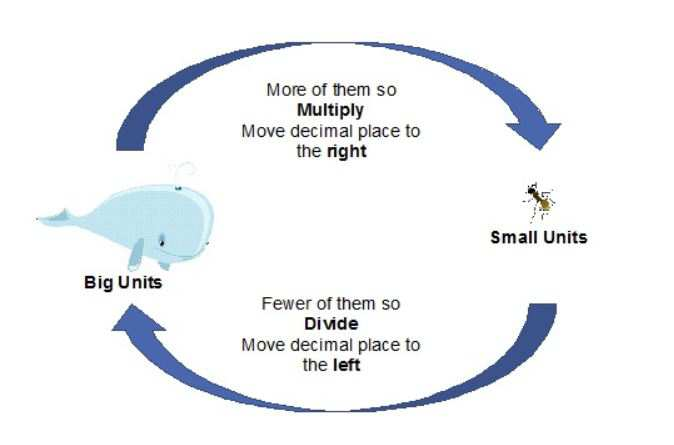The main units used are those that are a thousand times bigger or smaller.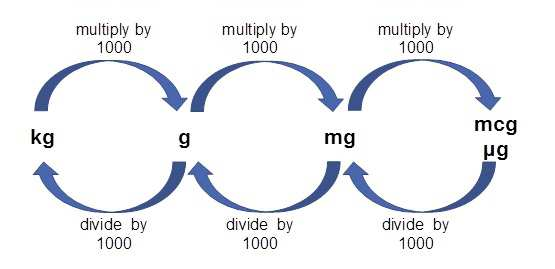We could replace grams in the diagram with litres, metres or moles. The same prefixes would be used.

#### Example 1

Express 5mg in micrograms.

Solution

To convert milligrams to micrograms we need to multiply by 1000.
As we are dealing with a whole number, this would involve adding three zeros on the end.

5 mg = 5 × 1000 = 5000 μg or 5000 mcg

#### Example 2

Express 0.5g in milligrams.

Solution

To convert grams to milligrams we need to multiply by 1000.

As we are dealing with a decimal value, multiplying by a thousand would involve moving the decimal place three places to the right.

Let’s add some zeros on the end so we have somewhere to move the decimal point to.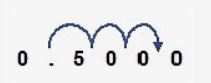The last zero isn’t needed as it comes after the decimal point.

So 0.5 g = 0.5 × 1000 = 500 mg

#### Example 3

Express 300ml in litres.

Solution

To convert millilitres to litres we need to divide by 1000.

This involves moving the decimal place three places to the left.

Let’s add some zeros on the left to make sure we have somewhere to move the decimal point to.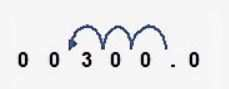The first and last zeros are not needed as the decimal point ends up after the second zero.

So 300 ml = 300 ÷ 1000 = 0.3 L

#### Example 4

A patient is ordered 1.2g of a medicine. The medication is available in 400mg tablets. How many tablets will you administer?

Solution

Notice that in this example, the units for the required dose are different to that of the stock dose.

If we convert the required dose from grams to milligrams, then 1.2g = 1200 mg

The number of tablets required is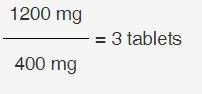### Exercise 1

Express 0.75 grams in milligrams

#### Solution

0.75 g × 1000 = 750 mg

### Exercise 2

Express 500 grams in kilograms

#### Solution

500 g ÷ 1000 = 0.5 kg

## Exercise 3

Express 56 milligrams in grams

#### Solution

56 mg ÷ 1000 = 0.056 g

### Exercise 4

Express 30 micrograms in milligrams

#### Solution

30 μg ÷ 1000 = 0.03 mg

### Exercise 5

Express 0.7 kilograms in grams

#### Solution

0.7 kg × 1000 = 700 g

### Exercise 6

Express 0.2 milligrams in micrograms

#### Solution

0.2 mg × 1000 = 200 μg

### Exercise 7

Express 300 cc in millilitres

300 cc = 300 ml

### Exercise 8

Express 700 millilitres in litres

#### Solution

700 ml ÷ 1000 = 0.7 L

### Exercise 9

Express 0.4 litres in millilitres

#### Solution

0.4 L × 1000 = 400 ml

### Exercise 10

Express 500 milligrams in grams

#### Solution

500 mg ÷ 1000 = 0.5 g

### Exercise 11

Express 1200 mg in grams

#### Solution

1200 mg ÷ 1000 = 1.2 g

### Exercise 12

Express 0.6 moles in millimoles

#### Solution

0.6 mol × 1000 = 600 mmol

### Exercise 13

Express 3.2 litres in millilitres

#### Solution

3.2 L × 1000 = 3200 ml

### Exercise 14

Express 50 millilitres in litres.

#### Solution

50 ml ÷ 1000 = 0.05 L

### Exercise 15

Express 450 µg in grams.

#### Solution

450 µg ÷ 1000 = 0.45 mg

0.45 mg ÷ 1000 = 0.00045 g